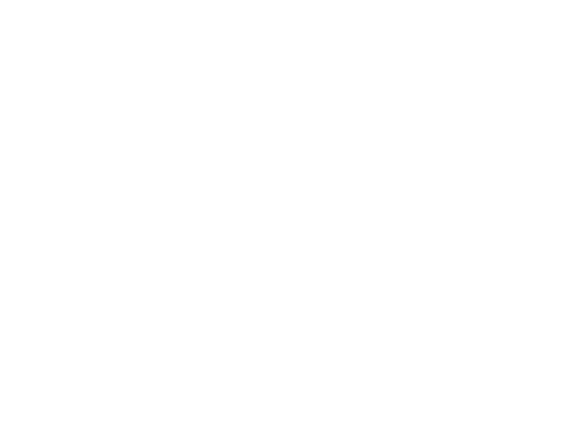Cody

# Problem 43610. nanoseconds passed today

Solution 1563827

Submitted on 19 Jun 2018 by Binbin Qi
This solution is locked. To view this solution, you need to provide a solution of the same size or smaller.

### Test Suite

Test Status Code Input and Output
1   Pass
x = '24-Oct-2016 20:26:28'; y_correct = 73587999999895.69; assert(abs(nanosecondsPassedToday(x)-y_correct)<0.05)

ans = 7.3588e+13

``
2   Pass
x = '24-Oct-2016 20:29:48'; y_correct = 73788000001385.8; assert(abs(nanosecondsPassedToday(x)-y_correct)<0.05)

ans = 7.3788e+13

``
3   Pass
x = '31-Oct-2016 13:19:42'; y_correct = 47982000002637.5; assert(abs(nanosecondsPassedToday(x)-y_correct)<0.05)

ans = 4.7982e+13

``
4   Pass
x = '04-Jan-1972 07:24:17'; y_correct = 26656999999657.3; assert(abs(nanosecondsPassedToday(x)-y_correct)<0.05)

ans = 2.6657e+13

``